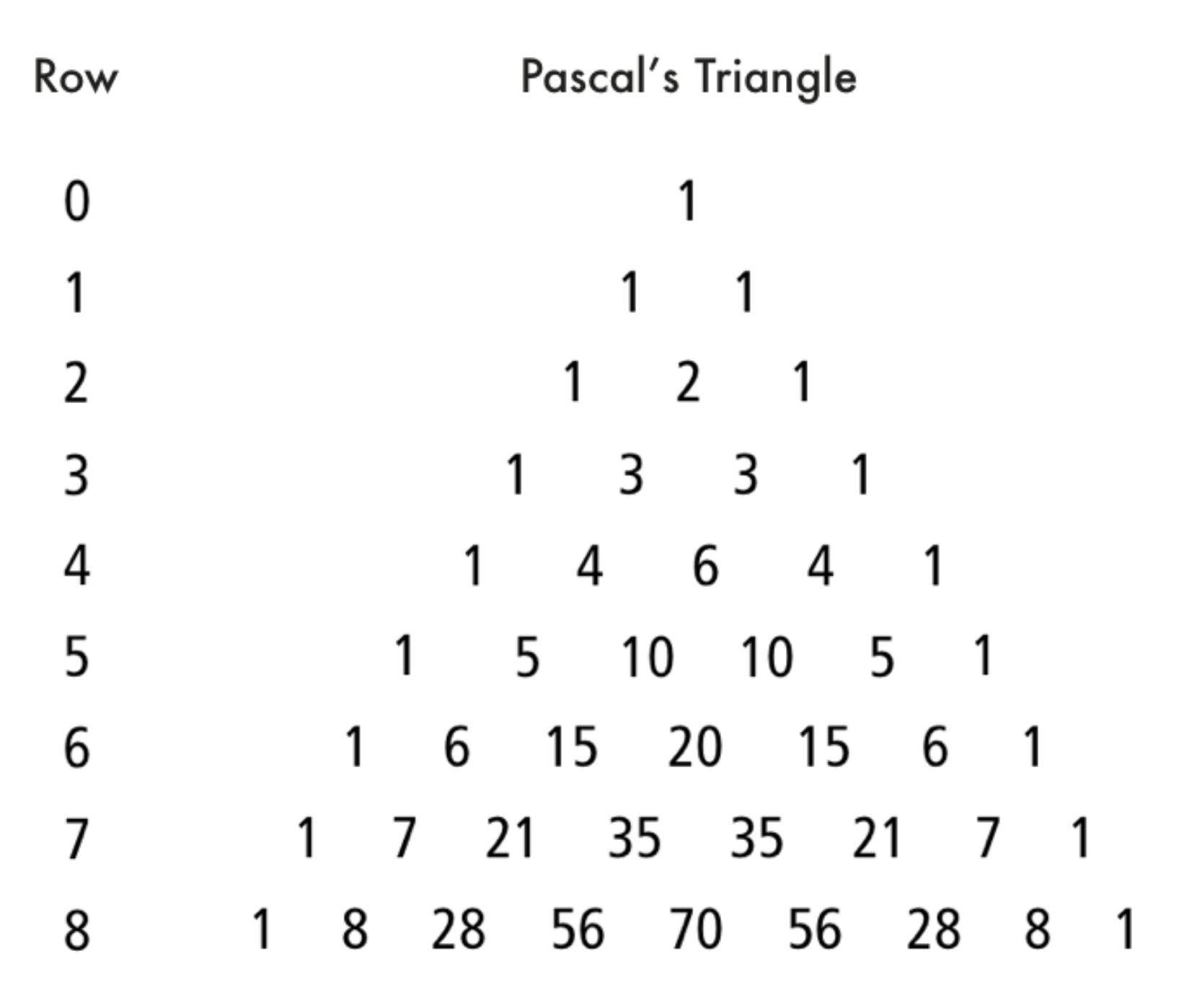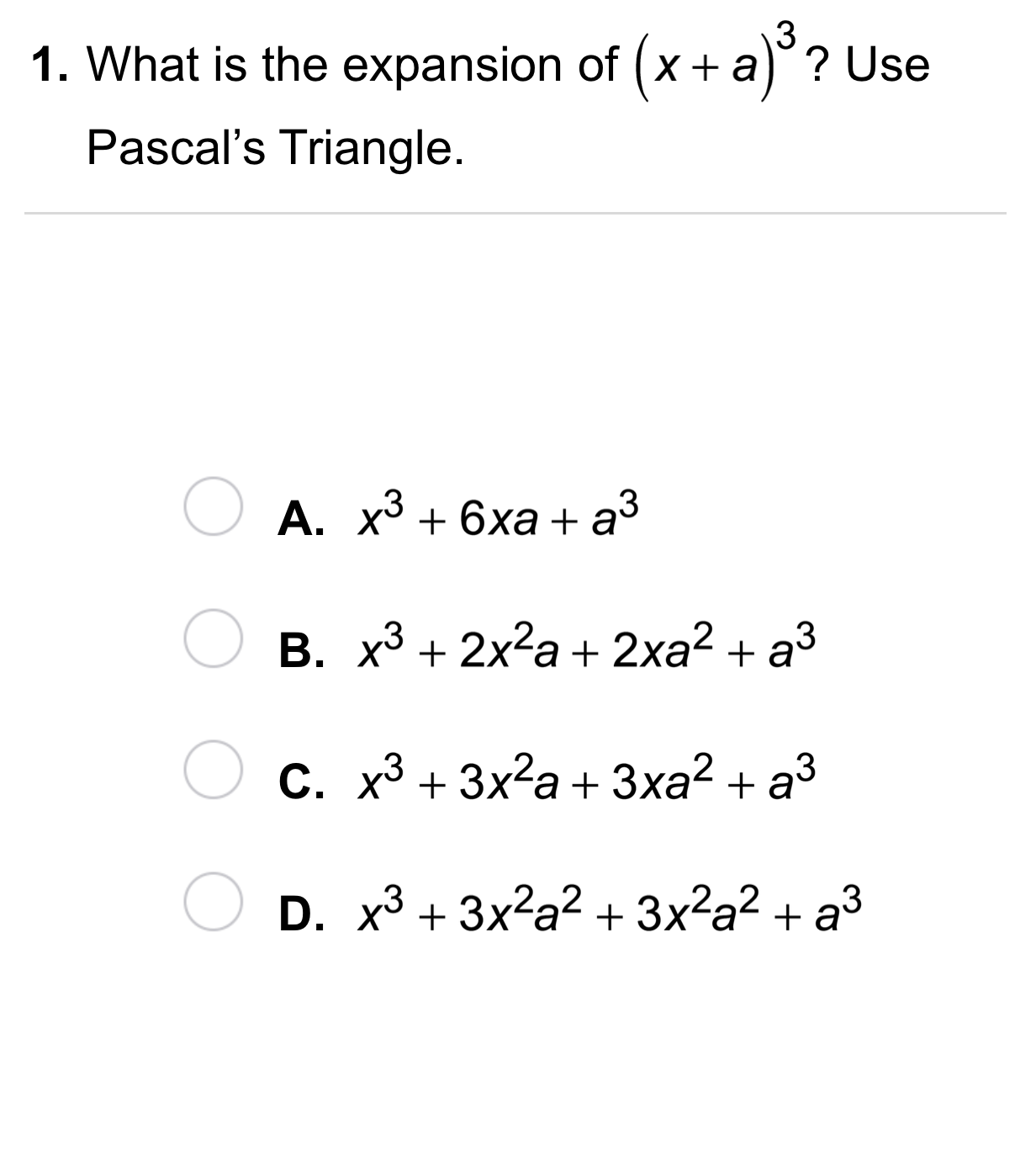Algebra 2 5-7 Complete Lesson: The Binomial Theorem
starstarstarstarstarstarstarstarstarstar
5 (1 rating)
by Matthew Richardson
| 22 Questions
Note from the author:
A complete formative lesson with embedded slideshow, mini lecture screencasts, checks for understanding, practice items, mixed review, and reflection. I create these assignments to supplement each lesson of Pearson's Common Core Edition Algebra 1, Algebra 2, and Geometry courses. See also mathquest.net and twitter.com/mathquestEDU.The outlined content above was added from outside of Formative.
1
10 pts
Solve It! How many unique letter combinations are possible using each of the following?
The same letters in different orders are one combination.

a. 2 of 5 letters b. 3 of 5 letters c. 2 of 6 letters d. 4 of 6 letters e. 3 of 6 letters

HINT: Use the diagram below, a previous response, or both.

Match each scenario on the left with its number of possible letter combinations on the right. Some categories may remain empty.
• a. 2 of 5 letters
• b. 3 of 5 letters
• c. 2 of 6 letters
• d. 4 of 6 letters
• e. 3 of 6 letters
• 5 letter combinations are possible
• 10 letter combinations are possible
• 15 letter combinations are possible
• 20 letter combinations are possible2
2
10 pts
Problem 1 Got It?
A
B
C
D3
10 pts
Problem 2 Got It? What is the expansion of the expression? Use the Binomial Theorem.4
4
10 pts
A
B
C
D5
5
10 pts
A
B
C
D6
6
10 pts
A
B
C
D7
7
10 pts
A
B
C
D8
10 pts
Vocabulary: Tell whether the expression can be expanded using the Binomial Theorem.

Yes, the expression can be expanded using the Binomial Theorem.
No, the expression can not be expanded using the Binomial Theorem.
9
10 pts
Vocabulary: Tell whether the expression can be expanded using the Binomial Theorem.

Yes, the expression can be expanded using the Binomial Theorem.
No, the expression can not be expanded using the Binomial Theorem.
10
10 pts
Vocabulary: Tell whether the expression can be expanded using the Binomial Theorem.

No, the expression can not be expanded using the Binomial Theorem.
Yes, the expression can be expanded using the Binomial Theorem.
12
10 pts
Reasoning: Using Pascal's Triangle, determine the number of terms in the expansion of the expression below.

11
12
13
10
13
10 pts
Reasoning: How many terms are there in the expansion of the expression below?

n + 1
2n
n
n - 214
10 pts
Review Lesson 5-6: Identify all of the roots of the polynomial equation.
• 0
• -1
• 3

• -3

15
10 pts
Review Lesson 4-8: Simplify the expression.

16
10 pts
Review Lesson 4-8: Simplify the expression.

17
10 pts
Review Lesson 4-8: Simplify the expression.18
10 pts
Vocabulary Review: Identify the next number in each pattern.
• 1
• 40
• 13
• 50
• 70
• 9
• 11
• 2
• 1, 2, 1, 2, 1,...
• 1, 3, 5, 7, 9,...
• 100, 90, 80, 70, 60,...19
10 pts
Use Your Vocabulary: Identify whether each sentence shows the correct use of expand.
• The writer expanded the short story into a novel.
• Heat expands most metals.
• The balloon expanded as all of its air was let out.
• The expanded form of (3- 4)² is

• Correct use of expand
• Not a correct use of expand
20
10 pts
Use Your Vocabulary: The expression is the expansion of which power of a binomial?

(5 - x
5² -
(5 + x
(25 - x
21
100 pts
Notes: Take a clear picture or screenshot of your Cornell notes for this lesson. Upload it to the canvas. Zoom and pan as needed.

For a refresher on the Cornell note-taking system, click here.
22
10 pts
Reflection: Math Success
Add to my formatives list

Formative uses cookies to allow us to better understand how the site is used. By continuing to use this site, you consent to the Terms of Service and Privacy Policy.